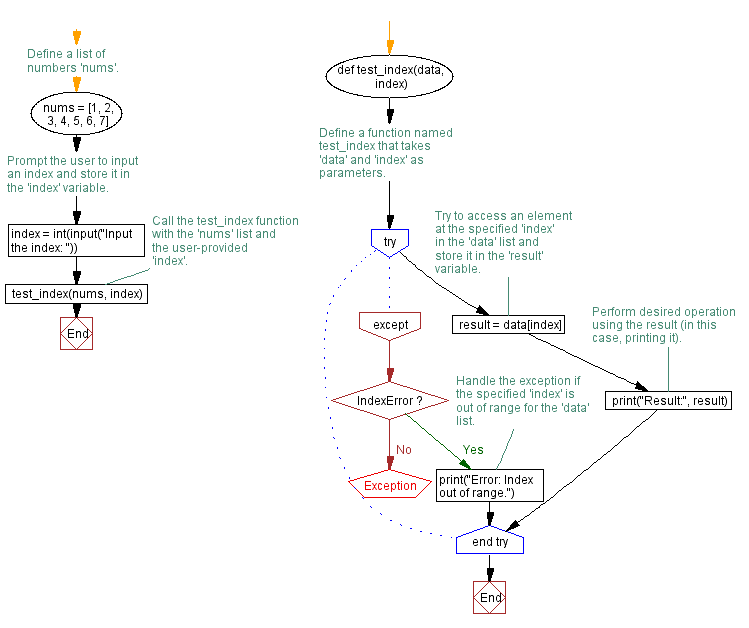﻿ Python program for Handling IndexError

# Handling IndexError Exception in Python list operation program

## Python Exception Handling: Exercise-6 with Solution

Write a Python program that executes an operation on a list and handles an IndexError exception if the index is out of range.

exception IndexError:

Raised when a sequence subscript is out of range. (Slice indices are silently truncated to fall in the allowed range; if an index is not an integer, TypeError is raised.)

Sample Solution:

Code:

``````# Define a function named test_index that takes 'data' and 'index' as parameters.
def test_index(data, index):
try:
# Try to access an element at the specified 'index' in the 'data' list and store it in the 'result' variable.
result = data[index]
# Perform desired operation using the result (in this case, printing it).
print("Result:", result)
except IndexError:
# Handle the exception if the specified 'index' is out of range for the 'data' list.
print("Error: Index out of range.")

# Define a list of numbers 'nums'.
nums = [1, 2, 3, 4, 5, 6, 7]
# Prompt the user to input an index and store it in the 'index' variable.
index = int(input("Input the index: "))
# Call the test_index function with the 'nums' list and the user-provided 'index'.
test_index(nums, index)
``````

Explanation:

In the above exercise,

• The "perform_operation()" function takes two parameters: data, which represents the list, and index, which represents the index of the element.
• Inside the function, we use a try block to access the element at the specified index in the list. If the index is within the list range, the element is assigned to the result variable.
• We can then execute the desired operation using the result. In this example, we simply print the result.
• If the index is out of range and an IndexError exception occurs, the program jumps to the except block.
• In the except block, we catch the IndexError exception and print an error message indicating that the index is out of range.

Output:

```Input the index: 0
Result: 1
```
```Input the index: 4
Result: 5
```
```Input the index: 9
Error: Index out of range.
```

Flowchart:What is the difficulty level of this exercise?

Test your Programming skills with w3resource's quiz.

﻿